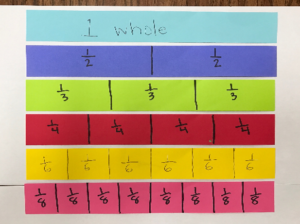# Proving Equivalent Fractions with Models

In 3rd grade, students are expected to generate simple equivalent fractions.

Example: 1/2 = 4/8 and 4/6 = 2/3.

Models should be used to help students identify equivalent fractions. Sentence strips or strips of paper cut into congruent rectangles can be used to model the idea of equivalency.Students will build conceptual understanding of equivalent fractions through the use of area and linear models. Number lines should be often used to model as it is an efficient strategy for finding equivalent fractions.As the standard states, students should be able to “explain why fractions are equivalent e.g, by using a visual fractional model”. The use of procedures or algorithms is not a 3rd grade expectation. Students will have more success and understanding when they can prove if two fractions are equal or not.

Learn Zillion – Recognize Equivalent Fractions Using Number Lines: https://learnzillion.com/lesson_plans/4739-recognize-equivalent-fractions-using-number-lines

Comparing using a number line –